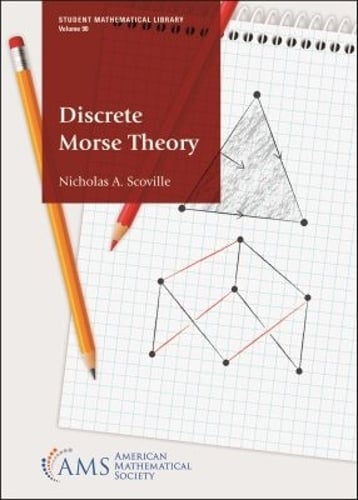•# Discrete Morse Theory - Student Mathematical Library (Paperback)

(author)
£52.95
Paperback 273 Pages / Published: 30/11/2019
• We can order this

Usually dispatched within 2 weeks

Discrete Morse theory is a powerful tool combining ideas in both topology and combinatorics. Invented by Robin Forman in the mid 1990s, discrete Morse theory is a combinatorial analogue of Marston Morse's classical Morse theory. Its applications are vast, including applications to topological data analysis, combinatorics, and computer science.

This book, the first one devoted solely to discrete Morse theory, serves as an introduction to the subject. Since the book restricts the study of discrete Morse theory to abstract simplicial complexes, a course in mathematical proof writing is the only prerequisite needed. Topics covered include simplicial complexes, simple homotopy, collapsibility, gradient vector fields, Hasse diagrams, simplicial homology, persistent homology, discrete Morse inequalities, the Morse complex, discrete Morse homology, and strong discrete Morse functions. Students of computer science will also find the book beneficial as it includes topics such as Boolean functions, evasiveness, and has a chapter devoted to some computational aspects of discrete Morse theory. The book is appropriate for a course in discrete Morse theory, a supplemental text to a course in algebraic topology or topological combinatorics, or an independent study.

Publisher: American Mathematical Society
ISBN: 9781470452988
Number of pages: 273
Dimensions: 216 x 140 mm